# 第三十三节，目标检测之选择性搜索-Selective Search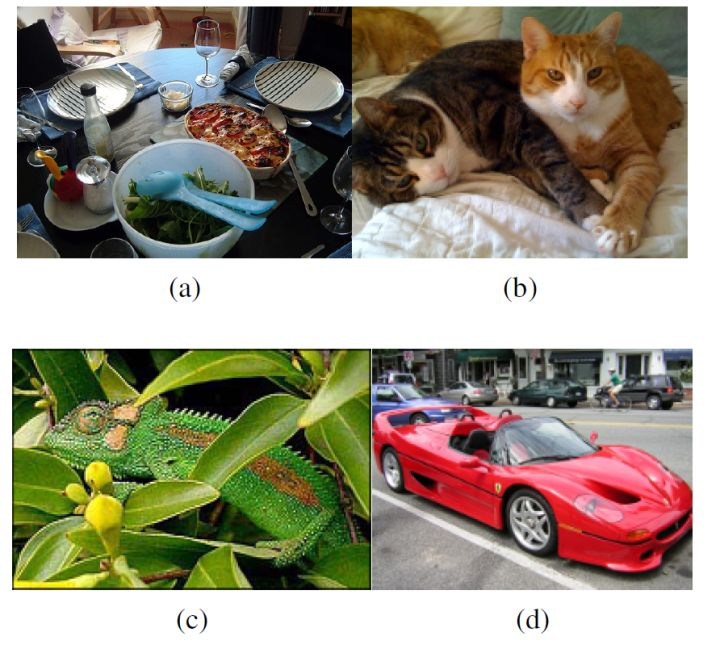selective search的策略是，既然是不知道尺度是怎样的，那我们就尽可能遍历所有的尺度好了，但是不同于暴力穷举，我们可以先利用基于图的图像分割的方法得到小尺度的区域，然后一次次合并得到大的尺寸就好了，这样也符合人类的视觉认知。既然特征很多，那就把我们知道的特征都用上，但是同时也要照顾下计算复杂度，不然和穷举法也没啥区别了。最后还要做的是能够对每个区域进行排序，这样你想要多少个候选我就产生多少个，不然总是产生那么多你也用不完不是吗？

•  适应不同尺度（Capture All Scales）：穷举搜索（Exhaustive Selective）通过改变窗口大小来适应物体的不同尺度，选择搜索（Selective Search）同样无法避免这个问题。算法采用了图像分割（Image Segmentation）以及使用一种层次算法（Hierarchical Algorithm）有效地解决了这个问题。
• 多样化（Diversification）：单一的策略无法应对多种类别的图像。使用颜色（color）、纹理（texture）、大小（size）等多种策略对分割好的区域（region）进行合并。
• 速度快（Fast to Compute）：算法，就像功夫一样，唯快不破！

### 一 选择性搜索的具体算法（区域合并算法）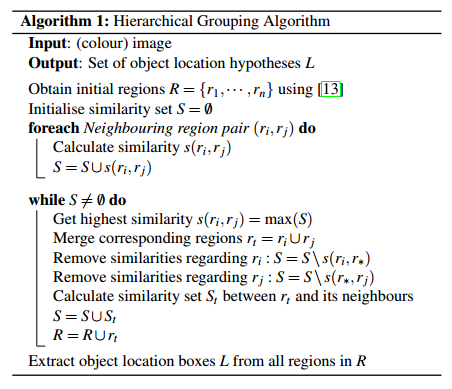输入: 一张图片

1: 利用切分方法得到候选的区域集合R = {r1,r2,…,rn}
2: 初始化相似集合S = ϕ
3: foreach 遍历邻居区域对(ri,rj) do
4:     计算相似度s(ri,rj)
5:     S = S  ∪ s(ri,rj)
6: while S not=ϕ do
7:     从S中得到最大的相似度s(ri,rj)=max(S)
8:     合并对应的区域rt = ri ∪ rj
9:     移除ri对应的所有相似度：S = S\s(ri,r*)
10:    移除rj对应的所有相似度：S = S\s(r*,rj)
11:    计算rt对应的相似度集合St
12:    S = S ∪ St
13:    R = R ∪ rt
14: L = R中所有区域对应的边框

### 二 保持多样性的策略

• 多种颜色空间，考虑RGB、灰度、HSV及其变种等
• 多种相似度度量标准，既考虑颜色相似度，又考虑纹理、大小、重叠情况等。
• 通过改变阈值初始化原始区域，阈值越大，分割的区域越少。

#### 1、颜色空间变换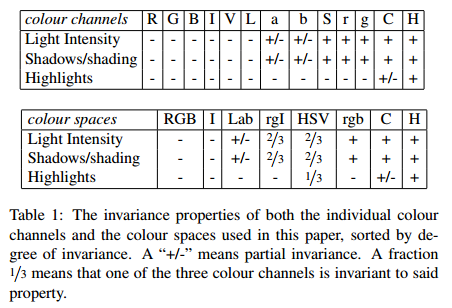• #### 颜色相似度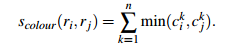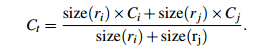• 纹理相似度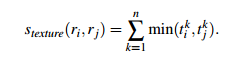• 优先合并小的区域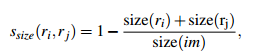• 区域的合适度距离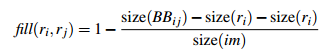• 合并上面四种相似度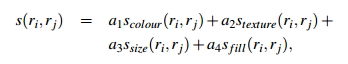### 四 选择性搜索性能评估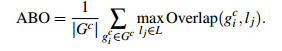#### 1、单一策略评估

• 使用RGB色彩空间(基于图的图像分割会利用不同的色彩进行图像区域分割)
• 采用四种相似度计算的组合方式
• 设置图像分割的阈值k=50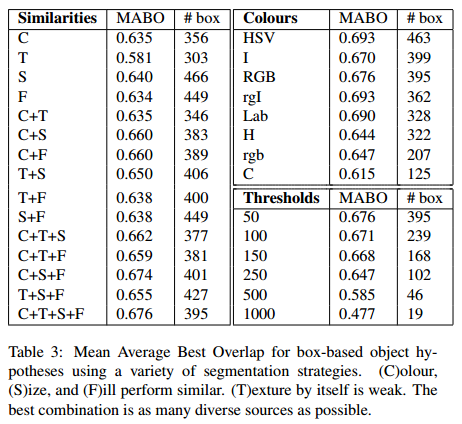#### 2、多样性策略组合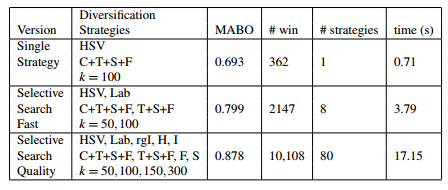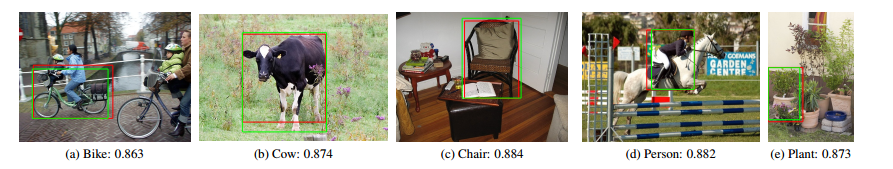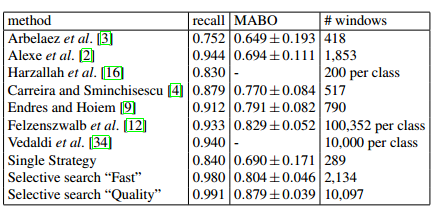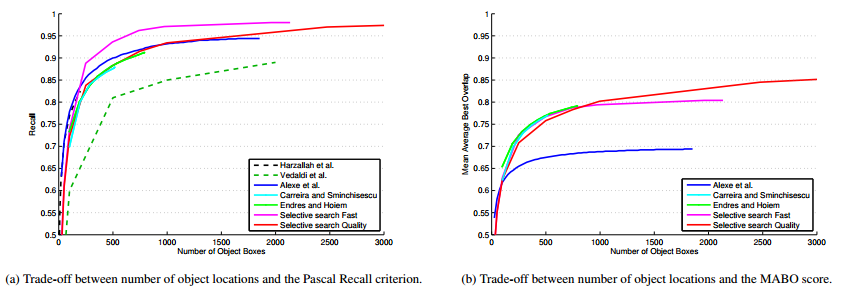### 五、代码实现

我们可以通过下面命令直接安装Selective Search包。

pip install selectivesearch

# -*- coding: utf-8 -*-
from __future__ import (
division,
print_function,
)

import skimage.data
import matplotlib.pyplot as plt
import matplotlib.patches as mpatches
import selectivesearch
import numpy as np

def main():

# 加载图片数据
img = skimage.data.astronaut()

'''
执行selective search，regions格式如下
[
{
'rect': (left, top, width, height),
'labels': [...],
'size': component_size
},
...
]
'''
img_lbl, regions = selectivesearch.selective_search(
img, scale=500, sigma=0.9, min_size=10)

#计算一共分割了多少个原始候选区域
temp = set()
for i in range(img_lbl.shape):
for j in range(img_lbl.shape):
print(len(temp))       #286

#计算利用Selective Search算法得到了多少个候选区域
print(len(regions))    #570
#创建一个集合 元素不会重复，每一个元素都是一个list(左上角x，左上角y,宽,高)，表示一个候选区域的边框
candidates = set()
for r in regions:
#排除重复的候选区
if r['rect'] in candidates:
continue
#排除小于 2000 pixels的候选区域(并不是bounding box中的区域大小)
if r['size'] < 2000:
continue
#排除扭曲的候选区域边框  即只保留近似正方形的
x, y, w, h = r['rect']
if w / h > 1.2 or h / w > 1.2:
continue

#在原始图像上绘制候选区域边框
fig, ax = plt.subplots(ncols=1, nrows=1, figsize=(6, 6))
ax.imshow(img)
for x, y, w, h in candidates:
print(x, y, w, h)
rect = mpatches.Rectangle(
(x, y), w, h, fill=False, edgecolor='red', linewidth=1)

plt.show()

if __name__ == "__main__":
main()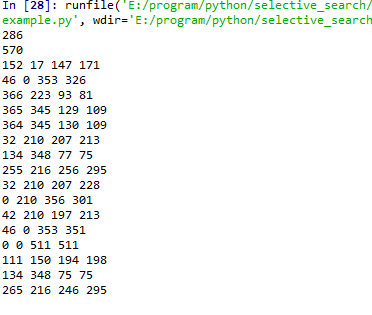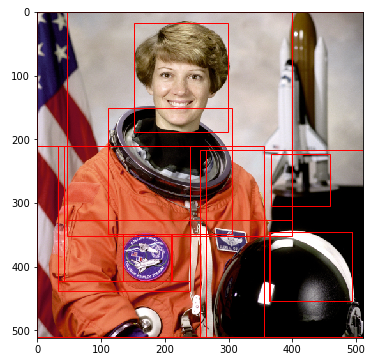selective_search函数的定义如下：

def selective_search(
im_orig, scale=1.0, sigma=0.8, min_size=50):
'''Selective Search

首先通过基于图的图像分割方法初始化原始区域，就是将图像分割成很多很多的小块
然后我们使用贪心策略，计算每两个相邻的区域的相似度
然后每次合并最相似的两块，直到最终只剩下一块完整的图片
然后这其中每次产生的图像块包括合并的图像块我们都保存下来

Parameters
----------
im_orig : ndarray
Input image
scale : int
Free parameter. Higher means larger clusters in felzenszwalb segmentation.
sigma : float
Width of Gaussian kernel for felzenszwalb segmentation.
min_size : int
Minimum component size for felzenszwalb segmentation.
Returns
-------
img : ndarray
image with region label
region label is stored in the 4th value of each pixel [r,g,b,(region)]
regions : array of dict
[
{
'rect': (left, top, width, height),
'labels': [...],
'size': component_size 候选区域大小，并不是边框的大小
},
...
]
'''
assert im_orig.shape == 3, "3ch image is expected"

# load image and get smallest regions
# region label is stored in the 4th value of each pixel [r,g,b,(region)]
#图片分割 把候选区域标签合并到最后一个通道上 height x width x 4  每一个像素的值为[r,g,b,(region)]
img = _generate_segments(im_orig, scale, sigma, min_size)

if img is None:
return None, {}

#计算图像大小
imsize = img.shape * img.shape

#dict类型，键值为候选区域的标签   值为候选区域的信息，包括候选区域的边框，以及区域的大小，颜色直方图，纹理特征直方图等信息
R = _extract_regions(img)

#list类型 每一个元素都是邻居候选区域对(ri,rj)  (即两两相交的候选区域)
neighbours = _extract_neighbours(R)

# calculate initial similarities 初始化相似集合S = ϕ
S = {}
#计算每一个邻居候选区域对的相似度s(ri,rj)
for (ai, ar), (bi, br) in neighbours:
#S=S∪s(ri,rj)  ai表示候选区域ar的标签  比如当ai=1 bi=2 S[(1,2)就表示候选区域1和候选区域2的相似度
S[(ai, bi)] = _calc_sim(ar, br, imsize)

# hierarchal search 层次搜索 直至相似度集合为空
while S != {}:

# get highest similarity  获取相似度最高的两个候选区域  i,j表示候选区域标签
i, j = sorted(S.items(), key=lambda i: i)[-1]   #按照相似度排序

# merge corresponding regions  合并相似度最高的两个邻居候选区域 rt = ri∪rj ,R = R∪rt
t = max(R.keys()) + 1.0
R[t] = _merge_regions(R[i], R[j])

# mark similarities for regions to be removed   获取需要删除的元素的键值
key_to_delete = []
for k, v in S.items():       #k表示邻居候选区域对(i,j)  v表示候选区域(i,j)表示相似度
if (i in k) or (j in k):
key_to_delete.append(k)

# remove old similarities of related regions 移除候选区域ri对应的所有相似度：S = S\s(ri,r*)  移除候选区域rj对应的所有相似度：S = S\s(r*,rj)
for k in key_to_delete:
del S[k]

# calculate similarity set with the new region  计算候选区域rt对应的相似度集合St,S = S∪St
for k in filter(lambda a: a != (i, j), key_to_delete):
n = k if k in (i, j) else k
S[(t, n)] = _calc_sim(R[t], R[n], imsize)

#获取每一个候选区域的的信息  边框、以及候选区域size,标签
regions = []
for k, r in R.items():
regions.append({
'rect': (
r['min_x'], r['min_y'],
r['max_x'] - r['min_x'], r['max_y'] - r['min_y']),
'size': r['size'],
'labels': r['labels']
})

#img：基于图的图像分割得到的候选区域   regions：Selective Search算法得到的候选区域
return img, regions# -*- coding: utf-8 -*-
import skimage.io
import skimage.feature
import skimage.color
import skimage.transform
import skimage.util
import skimage.segmentation
import numpy

# "Selective Search for Object Recognition" by J.R.R. Uijlings et al.
#
#  - Modified version with LBP extractor for texture vectorization

def _generate_segments(im_orig, scale, sigma, min_size):
"""
segment smallest regions by the algorithm of Felzenswalb and
Huttenlocher
"""

# open the Image   segment_mask : (width, height) ndarray  Integer mask indicating segment labels.
skimage.util.img_as_float(im_orig), scale=scale, sigma=sigma,
min_size=min_size)

# merge mask channel to the image as a 4th channel 把类别合并到最后一个通道上 height x width x 4
im_orig = numpy.append(
im_orig, numpy.zeros(im_orig.shape[:2])[:, :, numpy.newaxis], axis=2)

return im_orig

def _sim_colour(r1, r2):
"""
计算颜色相似度

calculate the sum of histogram intersection of colour

args:
r1：候选区域r1
r2：候选区域r2

return：[0,3]之间的数值

"""
return sum([min(a, b) for a, b in zip(r1["hist_c"], r2["hist_c"])])

def _sim_texture(r1, r2):
"""
计算纹理特征相似度

calculate the sum of histogram intersection of texture

args:
r1：候选区域r1
r2：候选区域r2

return：[0,3]之间的数值

"""
return sum([min(a, b) for a, b in zip(r1["hist_t"], r2["hist_t"])])

def _sim_size(r1, r2, imsize):
"""
计算候选区域大小相似度

calculate the size similarity over the image

args:
r1：候选区域r1
r2：候选区域r2

return：[0,1]之间的数值

"""
return 1.0 - (r1["size"] + r2["size"]) / imsize

def _sim_fill(r1, r2, imsize):
"""
计算候选区域的距离合适度相似度

calculate the fill similarity over the image

args:
r1：候选区域r1
r2：候选区域r2
imsize：原图像像素数

return：[0,1]之间的数值

"""
bbsize = (
(max(r1["max_x"], r2["max_x"]) - min(r1["min_x"], r2["min_x"]))
* (max(r1["max_y"], r2["max_y"]) - min(r1["min_y"], r2["min_y"]))
)
return 1.0 - (bbsize - r1["size"] - r2["size"]) / imsize

def _calc_sim(r1, r2, imsize):
'''
计算两个候选区域的相似度，权重系数默认都是1

args:
r1：候选区域r1
r2：候选区域r2
imsize：原图片像素数
'''
return (_sim_colour(r1, r2) + _sim_texture(r1, r2)
+ _sim_size(r1, r2, imsize) + _sim_fill(r1, r2, imsize))

def _calc_colour_hist(img):
"""
使用L1-norm归一化获取图像每个颜色通道的25 bins的直方图，这样每个区域都可以得到一个75维的向量

calculate colour histogram for each region

the size of output histogram will be BINS * COLOUR_CHANNELS(3)

number of bins is 25 as same as [uijlings_ijcv2013_draft.pdf]

extract HSV

args:
img：ndarray类型， 形状为候选区域像素数 x 3(h,s,v)

return：一维的ndarray类型，长度为75

"""

BINS = 25
hist = numpy.array([])

for colour_channel in (0, 1, 2):

# extracting one colour channel
c = img[:, colour_channel]

# calculate histogram for each colour and join to the result
#计算每一个颜色通道的25 bins的直方图 然后合并到一个一维数组中
hist = numpy.concatenate(
[hist] + [numpy.histogram(c, BINS, (0.0, 255.0))])

# L1 normalize  len(img):候选区域像素数
hist = hist / len(img)

return hist

"""
原文：对每个颜色通道的8个不同方向计算方差σ=1的高斯微分（Gaussian Derivative，这里使用LBP替代

calculate texture gradient for entire image

The original SelectiveSearch algorithm proposed Gaussian derivative
for 8 orientations, but we use LBP instead.

output will be [height(*)][width(*)]

args：
img： ndarray类型，形状为height x width x 4，每一个像素的值为 [r,g,b,(region)]

return：纹理特征，形状为height x width x 4
"""
ret = numpy.zeros((img.shape, img.shape, img.shape))

for colour_channel in (0, 1, 2):
ret[:, :, colour_channel] = skimage.feature.local_binary_pattern(
img[:, :, colour_channel], 8, 1.0)

return ret

def _calc_texture_hist(img):
"""
使用L1-norm归一化获取图像每个颜色通道的每个方向的10 bins的直方图，这样就可以获取到一个240（10x8x3）维的向量

calculate texture histogram for each region

calculate the histogram of gradient for each colours
the size of output histogram will be
BINS * ORIENTATIONS * COLOUR_CHANNELS(3)

args:
img：候选区域纹理特征   形状为候选区域像素数 x 4(r,g,b,(region))

return：一维的ndarray类型，长度为240
"""
BINS = 10

hist = numpy.array([])

for colour_channel in (0, 1, 2):

# mask by the colour channel
fd = img[:, colour_channel]

# calculate histogram for each orientation and concatenate them all
# and join to the result
hist = numpy.concatenate(
[hist] + [numpy.histogram(fd, BINS, (0.0, 1.0))])

# L1 Normalize   len(img):候选区域像素数
hist = hist / len(img)

return hist

def _extract_regions(img):
'''
提取每一个候选区域的信息   比如类别(region)为5的区域表示的是一只猫的选区，这里就是提取这只猫的边界框，左上角后右下角坐标

args:
img: ndarray类型，形状为height x width x 4，每一个像素的值为 [r,g,b,(region)]

return :
R:dict 每一个元素对应一个候选区域， 每个元素也是一个dict类型
{min_x:边界框的左上角x坐标,
min_y:边界框的左上角y坐标,
max_x:边界框的右下角x坐标,
max_y:边界框的右下角y坐标,
size:像素个数,
hist_c:颜色的直方图,
hist_t:纹理特征的直方图,}
'''
#保存所有候选区域的bounding box  每一个元素都是一个dict {最小x坐标值，最小y坐标值，最大x坐标值，最大y坐标值,类别}
#                            通过上面四个参数确定一个边界框
R = {}

# get hsv image   RGB转换为HSV色彩空间 height x width x 3
hsv = skimage.color.rgb2hsv(img[:, :, :3])

# pass 1: count pixel positions 遍历每一个像素
for y, i in enumerate(img):    #y = 0 -> height - 1

for x, (r, g, b, l) in enumerate(i):  # x = 0 -> height - 1

# initialize a new region
if l not in R:
R[l] = {
"min_x": 0xffff, "min_y": 0xffff,
"max_x": 0, "max_y": 0, "labels": [l]}

# bounding box
if R[l]["min_x"] > x:
R[l]["min_x"] = x
if R[l]["min_y"] > y:
R[l]["min_y"] = y
if R[l]["max_x"] < x:
R[l]["max_x"] = x
if R[l]["max_y"] < y:
R[l]["max_y"] = y

# pass 2: calculate texture gradient  纹理特征提取 利用LBP算子 height x width x 4

# pass 3: calculate colour histogram of each region  计算每一个候选区域(注意不是bounding box圈住的区域)的直方图
for k, v in R.items():

# colour histogram   height x width x 3 -> 候选区域k像素数 x 3（img[:, :, 3] == k返回的是一个二维坐标的集合）
masked_pixels = hsv[:, :, :][img[:, :, 3] == k]
R[k]["size"] = len(masked_pixels / 4)                  #候选区域k像素数
#在hsv色彩空间下，使用L1-norm归一化获取图像每个颜色通道的25 bins的直方图，这样每个区域都可以得到一个75维的向量

#在rgb色彩空间下，使用L1-norm归一化获取图像每个颜色通道的每个方向的10 bins的直方图，这样就可以获取到一个240（10x8x3）维的向量
R[k]["hist_t"] = _calc_texture_hist(tex_grad[:, :][img[:, :, 3] == k])   #tex_grad[:, :][img[:, :, 3] == k]形状为候选区域像素数 x 4

return R

def _extract_neighbours(regions):
'''
提取 邻居候选区域对(ri,rj)(即两两相交)

args:
regions：dict 每一个元素都对应一个候选区域
return：
返回一个list，每一个元素都对应一个邻居候选区域对
'''
#判断两个候选区域是否相交
def intersect(a, b):
if (a["min_x"] < b["min_x"] < a["max_x"]
and a["min_y"] < b["min_y"] < a["max_y"]) or (
a["min_x"] < b["max_x"] < a["max_x"]
and a["min_y"] < b["max_y"] < a["max_y"]) or (
a["min_x"] < b["min_x"] < a["max_x"]
and a["min_y"] < b["max_y"] < a["max_y"]) or (
a["min_x"] < b["max_x"] < a["max_x"]
and a["min_y"] < b["min_y"] < a["max_y"]):
return True
return False

#转换为list 每一个元素 (l,regions[l])
R = list(regions.items())
#保存两两相交候选区域对
neighbours = []
#每次抽取两个候选区域 两两组合，判断是否相交
for cur, a in enumerate(R[:-1]):
for b in R[cur + 1:]:
if intersect(a, b):
neighbours.append((a, b))

return neighbours

def _merge_regions(r1, r2):
'''
合并两个候选区域

args:
r1：候选区域1
r2：候选区域2

return：
返回合并后的候选区域rt
'''
new_size = r1["size"] + r2["size"]
rt = {
"min_x": min(r1["min_x"], r2["min_x"]),
"min_y": min(r1["min_y"], r2["min_y"]),
"max_x": max(r1["max_x"], r2["max_x"]),
"max_y": max(r1["max_y"], r2["max_y"]),
"size": new_size,
"hist_c": (
r1["hist_c"] * r1["size"] + r2["hist_c"] * r2["size"]) / new_size,
"hist_t": (
r1["hist_t"] * r1["size"] + r2["hist_t"] * r2["size"]) / new_size,
"labels": r1["labels"] + r2["labels"]
}
return rt

def selective_search(
im_orig, scale=1.0, sigma=0.8, min_size=50):
'''Selective Search

首先通过基于图的图像分割方法初始化原始区域，就是将图像分割成很多很多的小块
然后我们使用贪心策略，计算每两个相邻的区域的相似度
然后每次合并最相似的两块，直到最终只剩下一块完整的图片
然后这其中每次产生的图像块包括合并的图像块我们都保存下来

Parameters
----------
im_orig : ndarray
Input image
scale : int
Free parameter. Higher means larger clusters in felzenszwalb segmentation.
sigma : float
Width of Gaussian kernel for felzenszwalb segmentation.
min_size : int
Minimum component size for felzenszwalb segmentation.
Returns
-------
img : ndarray
image with region label
region label is stored in the 4th value of each pixel [r,g,b,(region)]
regions : array of dict
[
{
'rect': (left, top, width, height),
'labels': [...],
'size': component_size 候选区域大小，并不是边框的大小
},
...
]
'''
assert im_orig.shape == 3, "3ch image is expected"

# load image and get smallest regions
# region label is stored in the 4th value of each pixel [r,g,b,(region)]
#图片分割 把候选区域标签合并到最后一个通道上 height x width x 4  每一个像素的值为[r,g,b,(region)]
img = _generate_segments(im_orig, scale, sigma, min_size)

if img is None:
return None, {}

#计算图像大小
imsize = img.shape * img.shape

#dict类型，键值为候选区域的标签   值为候选区域的信息，包括候选区域的边框，以及区域的大小，颜色直方图，纹理特征直方图等信息
R = _extract_regions(img)

#list类型 每一个元素都是邻居候选区域对(ri,rj)  (即两两相交的候选区域)
neighbours = _extract_neighbours(R)

# calculate initial similarities 初始化相似集合S = ϕ
S = {}
#计算每一个邻居候选区域对的相似度s(ri,rj)
for (ai, ar), (bi, br) in neighbours:
#S=S∪s(ri,rj)  ai表示候选区域ar的标签  比如当ai=1 bi=2 S[(1,2)就表示候选区域1和候选区域2的相似度
S[(ai, bi)] = _calc_sim(ar, br, imsize)

# hierarchal search 层次搜索 直至相似度集合为空
while S != {}:

# get highest similarity  获取相似度最高的两个候选区域  i,j表示候选区域标签
i, j = sorted(S.items(), key=lambda i: i)[-1]   #按照相似度排序

# merge corresponding regions  合并相似度最高的两个邻居候选区域 rt = ri∪rj ,R = R∪rt
t = max(R.keys()) + 1.0
R[t] = _merge_regions(R[i], R[j])

# mark similarities for regions to be removed   获取需要删除的元素的键值
key_to_delete = []
for k, v in S.items():       #k表示邻居候选区域对(i,j)  v表示候选区域(i,j)表示相似度
if (i in k) or (j in k):
key_to_delete.append(k)

# remove old similarities of related regions 移除候选区域ri对应的所有相似度：S = S\s(ri,r*)  移除候选区域rj对应的所有相似度：S = S\s(r*,rj)
for k in key_to_delete:
del S[k]

# calculate similarity set with the new region  计算新的候选区域rt对应的相似度集合St,S = S∪St
for k in filter(lambda a: a != (i, j), key_to_delete):  #过滤除了(i,j)之外的候选区域
n = k if k in (i, j) else k
#计算新的候选区域t与候选区域n之间的相似度
S[(t, n)] = _calc_sim(R[t], R[n], imsize)

#获取每一个候选区域的的信息  边框、以及候选区域size,标签
regions = []
for k, r in R.items():
regions.append({
'rect': (
r['min_x'], r['min_y'],
r['max_x'] - r['min_x'], r['max_y'] - r['min_y']),
'size': r['size'],
'labels': r['labels']
})

#img：ndarray 基于图的图像分割得到的候选区域   regions：list Selective Search算法得到的候选区域
return img, regions
View Code

图像分割—基于图的图像分割（Graph-Based Image Segmentation）(附代码)

目标检测（1）-Selective Search